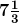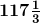# Prove the following

Question:

$117 \frac{1}{3}$ m long rope is cut into equal pieces measuringm each. How many such small pieces are these?

Solution:

From the question it is given that,

The length of the rope =m

= (117 × 3 + 1)/3

= 352/3m

Then length of each piece measures =m

= 22/3 m

So, the number of pieces of the rope = total length of the rope/ length of each piece

= (352/3)/ (22/3)

= (352/3) × (3/22)

= (16/1) × (1/1)

= 16

Hence, number of small pieces cut from them long rope is 16